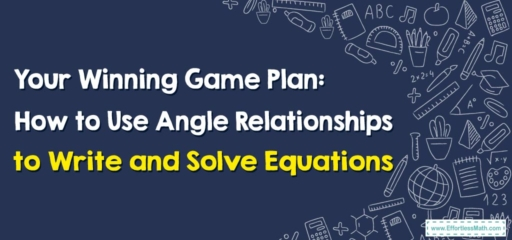# Your Winning Game Plan: How to Use Angle Relationships to Write and Solve Equations

Greetings, math team players!

In today's math match, we're tackling a big player: using angle relationships to write and solve equations. And just like any game, having the right strategies up your sleeve can lead you to a winning score. Let's break it down!## 1. Knowing Your Players: Angle Relationships

In this game, angle relationships are key players. Whether they’re complementary (adding up to 90 degrees), supplementary (adding up to $$180$$ degrees), vertical (opposite angles that are equal), or corresponding (angles in the same position in parallel lines), knowing your angles helps you strategize effectively.

## 2. The Game: Writing and Solving Equations

Our goal is to write equations that capture these angle relationships and solve them to find unknown angles.

### Your Winning Game Plan for Using Angle Relationships to Write and Solve Equations

Let’s dive into the game plan:

#### Step 1: Identify the Angle Relationships

Survey the field. What kind of angles are in play? Are they complementary, supplementary, vertical, or corresponding?

#### Step 2: Write the Equation

Using your knowledge of the angle relationships, write an equation. Remember, for complementary angles, the sum is $$90$$ degrees; for supplementary, it’s $$180$$ degrees. Vertical and corresponding angles are equal.

#### Step 3: Solve the Equation

Now, tackle that equation to find the value of the unknown angle.

Take this example: If we have two complementary angles, where one angle measures $$x$$ degrees and the other is $$25$$ degrees smaller, how can we find $$x$$?

1. Identify the Angle Relationships: The angles are complementary.
2. Write the Equation: Since they’re complementary, $$x + (x\ – 25) = 90$$.
3. Solve the Equation: Combining like terms, we get $$2x\ – 25 = 90$$. Adding $$25$$ to both sides gives us $$2x = 115$$, and dividing by $$2$$ gives us $$x = 57.5$$ degrees. Score!

And just like that, you’ve scored a win in this math match! With the right strategies, using angle relationships to write and solve equations becomes a game you’re always ready to play. Keep practicing, and remember, every math challenge is an opportunity to up your game!

### What people say about "Your Winning Game Plan: How to Use Angle Relationships to Write and Solve Equations - Effortless Math: We Help Students Learn to LOVE Mathematics"?

No one replied yet.

X
30% OFF

Limited time only!

Save Over 30%

SAVE $5 It was$16.99 now it is \$11.99